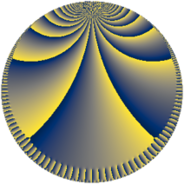# Properties

 Label 384.10.cLevel $384$ Weight $10$ Character orbit 384.c Rep. character $\chi_{384}(383,\cdot)$ Character field $\Q$ Dimension $144$ Sturm bound $640$

# Related objects

## Defining parameters

 Level: $$N$$ $$=$$ $$384 = 2^{7} \cdot 3$$ Weight: $$k$$ $$=$$ $$10$$ Character orbit: $$[\chi]$$ $$=$$ 384.c (of order $$2$$ and degree $$1$$) Character conductor: $$\operatorname{cond}(\chi)$$ $$=$$ $$12$$ Character field: $$\Q$$ Sturm bound: $$640$$

## Dimensions

The following table gives the dimensions of various subspaces of $$M_{10}(384, [\chi])$$.

Total New Old
Modular forms 592 144 448
Cusp forms 560 144 416
Eisenstein series 32 0 32

## Trace form

 $$144 q + O(q^{10})$$ $$144 q - 56250000 q^{25} - 75632 q^{33} - 778503024 q^{49} - 219408016 q^{57} - 887399712 q^{73} + 927683728 q^{81} - 4448121408 q^{97} + O(q^{100})$$

## Decomposition of $$S_{10}^{\mathrm{new}}(384, [\chi])$$ into newform subspaces

The newforms in this space have not yet been added to the LMFDB.

## Decomposition of $$S_{10}^{\mathrm{old}}(384, [\chi])$$ into lower level spaces

$$S_{10}^{\mathrm{old}}(384, [\chi]) \cong$$ $$S_{10}^{\mathrm{new}}(12, [\chi])$$$$^{\oplus 6}$$$$\oplus$$$$S_{10}^{\mathrm{new}}(48, [\chi])$$$$^{\oplus 4}$$$$\oplus$$$$S_{10}^{\mathrm{new}}(96, [\chi])$$$$^{\oplus 3}$$$$\oplus$$$$S_{10}^{\mathrm{new}}(192, [\chi])$$$$^{\oplus 2}$$